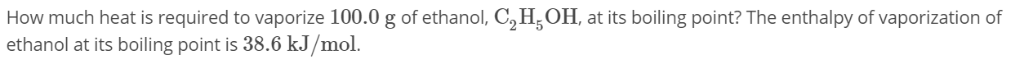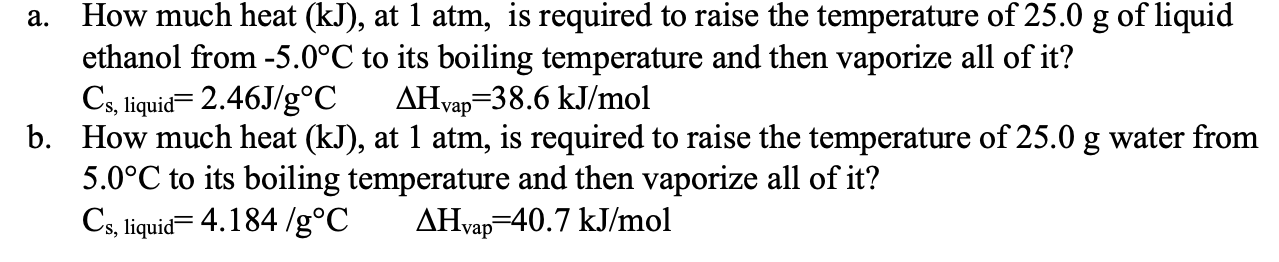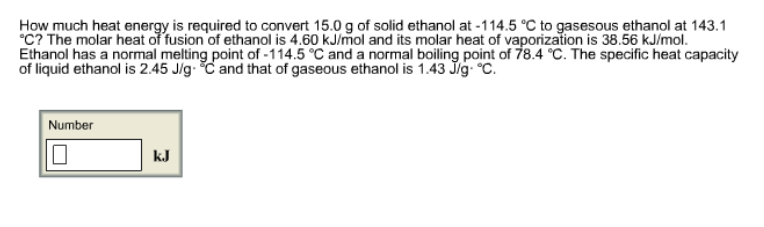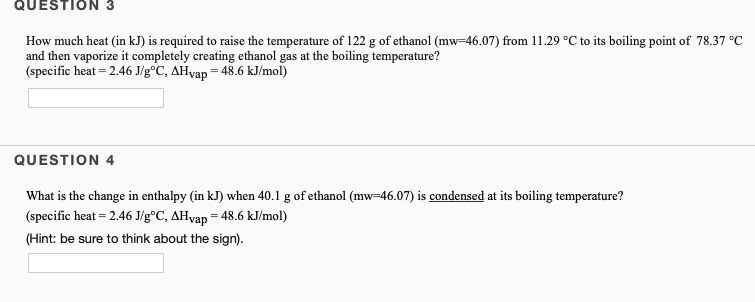# 222.The enthalpy of vaporization for chlorine is 20.41kJ/mol. How much heat is required to vaporize 293.39g...

222.The enthalpy of vaporization for chlorine is 20.41kJ/mol. How much heat is required to vaporize 293.39g of chlorine liquid at its boiling point? Report your answer in units of kJ.

This Homework Help Question: "222.The enthalpy of vaporization for chlorine is 20.41kJ/mol. How much heat is required to vaporize 293.39g..." No answers yet.

We need 10 more requests to produce the answer to this homework help question. Share with your friends to get the answer faster!

0 /10 have requested the answer to this homework help question.

Once 10 people have made a request, the answer to this question will be available in 1-2 days.
All students who have requested the answer will be notified once they are available.

#### Earn Coin

Coins can be redeemed for fabulous gifts.

Similar Homework Help Questions
• ### How much heat is required to vaporize 100.0 g of ethanol, C2H,OH, at its boiling point?...How much heat is required to vaporize 100.0 g of ethanol, C2H,OH, at its boiling point? The enthalpy of vaporization of ethanol at its boiling point is 38.6 kJ/mol.

• ### The enthalpy of fusion for methane (CH4) is 0.942kJ/mol. How much heat is required to melt...

The enthalpy of fusion for methane (CH4) is 0.942kJ/mol. How much heat is required to melt 37.91g of solid methane at its melting point? Report your answer in units of kJ.

• ### How much heat is required to vaporize 2.00 L of CCl4 at its normal boiling point?

The following data are given for . Normal melting point, -23C , normal boiling point, 77C ; density of liquid 1.59g/ml ;vapor pressure at 25C , 110 Torr .How much heat is required to vaporize 2.00 L of CCl4 at its normal boiling point?Answer in kJ

• ### a. How much heat (kJ), at 1 atm, is required to raise the temperature of 25.0...a. How much heat (kJ), at 1 atm, is required to raise the temperature of 25.0 g of liquid ethanol from -5.0°C to its boiling temperature and then vaporize all of it? Cs, liquid= 2.46J/g°C AHvap=38.6 kJ/mol b. How much heat (kJ), at 1 atm, is required to raise the temperature of 25.0 g water from 5.0°C to its boiling temperature and then vaporize all of it? Cs, liquid=4.184 /g°C AHvap=40.7 kJ/mol

• ### Determine the enthalpy of vaporization, in kJ/mol, of H₂O if 20.4 kJ of heat is needed...

Determine the enthalpy of vaporization, in kJ/mol, of H₂O if 20.4 kJ of heat is needed to vaporize 0.500 moles of H₂O.

• ### How much heat energy is required to convert 15.0 g of solid ethanol at -114.5 °C...How much heat energy is required to convert 15.0 g of solid ethanol at -114.5 °C to gasesous ethanol at 143.1 °C? The molar heat of fusion of ethanol is 4.60 kJ/mol and its molar heat of vaporization is 38.56 kJ/mol. Ethanol has a normal melting point of -114.5 °C and a normal boiling point of 78.4 °C. The specific heat capacity of liquid ethanol is 2.45 J/g·°C and that of gaseous ethanol is 1.43 J/g·°C. How much heat energy...

• ### How much energy is required to vaporize 158 g of butane (C4H10) at its boiling point,...

How much energy is required to vaporize 158 g of butane (C4H10) at its boiling point, if its ΔHvap is 24.3 kJ/mol

• ### QUESTION 3 How much heat (in kJ) is required to raise the temperature of 122 g of ethanol (mw 46.07) from 11.29 °C to i...QUESTION 3 How much heat (in kJ) is required to raise the temperature of 122 g of ethanol (mw 46.07) from 11.29 °C to its boiling point of 78.37 °C and then vaporize it completely creating ethanol gas at the boiling temperature? (specific heat - 2.46 J/g°C, AHyap = 48.6 kJ/mol) QUESTION 4 What is the change in enthalpy (in kJ) when 40.1 g of ethanol (mw-46.07) is condensed at its boiling temperature? (specific heat 2.46 J/g°C, AHvap 48.6 kJ/mol)...

• ### Butane (C4H10) has a heat of vaporization of 22.44 kJ/mol and a normal boiling point of...

Butane (C4H10) has a heat of vaporization of 22.44 kJ/mol and a normal boiling point of -0.4 ∘C. A 250 mL sealed flask contains 0.7 g of butane at −22∘C. How much butane is present as a liquid at −22∘C? Express your answer using two significant figures. If the butane is warmed to 25 ∘C, how much liquid butane is present?

• ### How much heat (in kJ) is required to raise the temperature of 202.8 g of ethanol...

How much heat (in kJ) is required to raise the temperature of 202.8 g of ethanol (mw=46.07) from 40.34 °C to its boiling point of  78.37 °C and then vaporize it completely creating ethanol gas at the boiling temperature? (specific heat = 2.46 J/g°C, ΔHvap = 48.6 kJ/mol)

Free Homework App